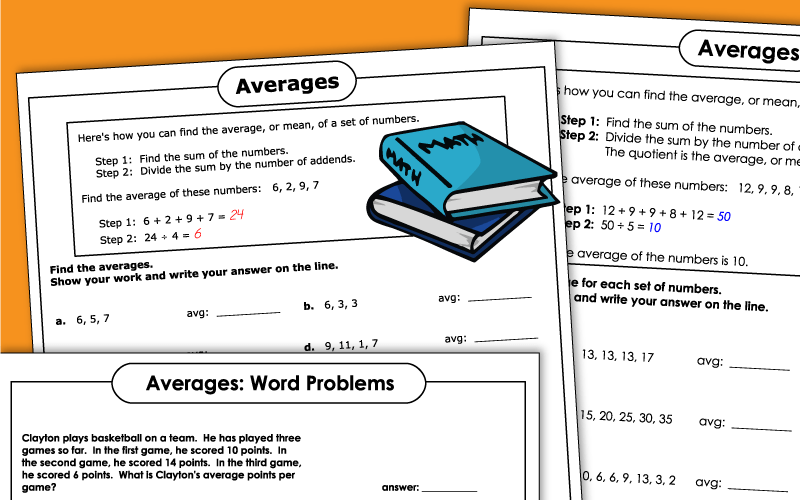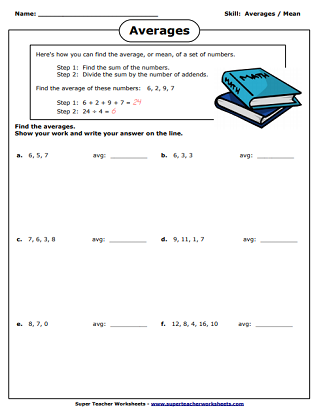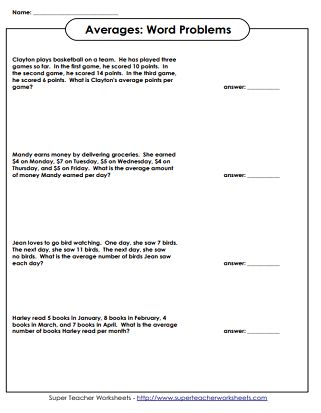# Math - Mean Averages

Printable worksheets for helping students learn about average/mean.  Some will require only basic division skills, while others may require knowledge of long division.## Basic Level(Basic Division Facts)

Find the average of the numbers shown.  Beginner's worksheet for finding mean.  (Requires only basic division skills.)
Word problems for finding the average number.  (Requires only basic division skills.)

## Intermediate Level(Long Division)

Calculate the average of the bowling scores.  (Requires long division.)
Find the average of the numbers shown.  (Requires long division.)

Mean, Median, Mode, and Range

Worksheets for calculating the mean, median, mode, and range for number lists.

## Worksheet PicturesMy Account
Site Information